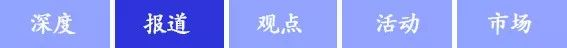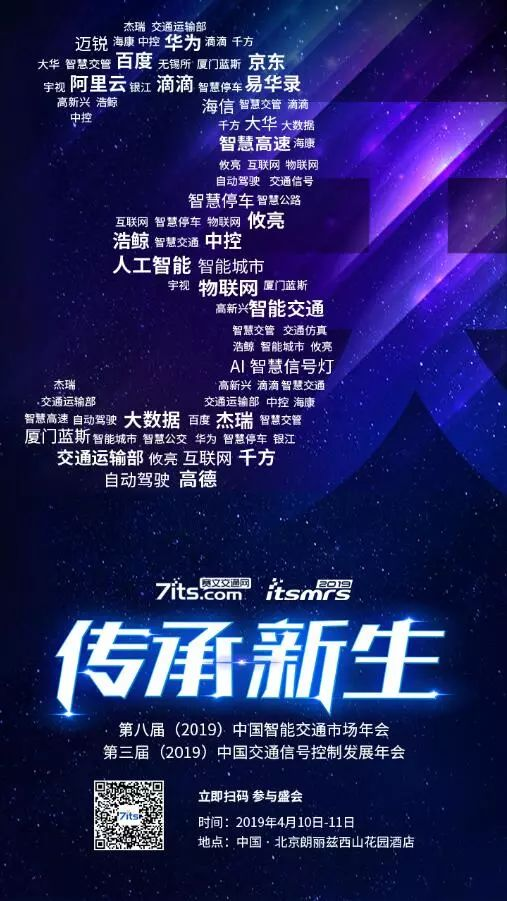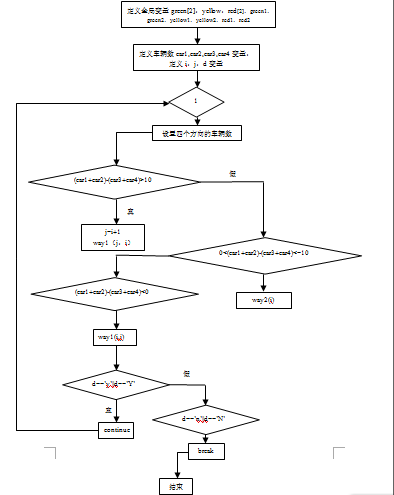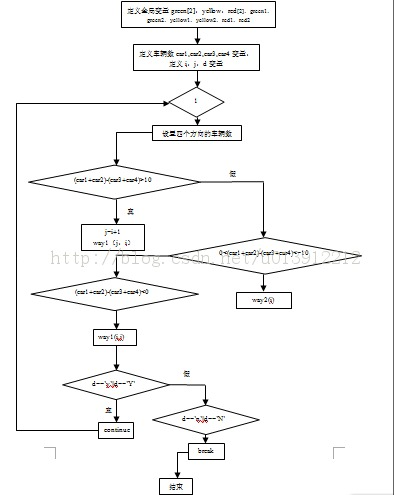• 基于质点假设的交叉口交通冲突严重程度量化，刘淼淼，邓成，鉴于传统的交通冲突研究分析的缺陷，提出基于质点单元假设的交叉口交通冲突严重程度量化模型。通过对视频图像序列进行计算机处理
• 交通冲突技术在平面交叉口安全评价中的应用，孙壮志，许奇，基于交通冲突技术定义和分类分析的基础上，本文提出严重冲突和非严重冲突判别原则，针对平面交叉口交通冲突类型，以四道口交叉口
• 信号交叉口通行能力计算方法探讨，张桂梅，李国文，介绍目前国内外常用的两种计算信号交叉口通行能力的方法(停车线法、冲突点法)，以某个具有代表性的信号交叉口为例，通过用两种方�
• 信号交叉口自行车对左转机动车饱和流率的影响研究，于泉，过雁鸣，为了分析信号交叉口自行车对左转机动车的冲突，本文主要以国内信号交叉口的自行车作为研究对象，研究自行车通过交叉口时不同的状
• 信控道路交叉口过街行人流影响下的右转车辆延误模型，李玲，李硕，交通延误是评价城市信控道路交叉口服务水平和车辆通行效率的一个重要指标。未设置右转专用相位的信号交叉口右转车辆与行人的冲突
• 论文研究-城市普通道路与快速路出口衔接区域信号交叉口公交优先实施方法.pdf, 针对城市普通道路与快速路出口衔接路段的公交优先实施方法开展研究，并提出一种针对该区域...
• 过滤算法 根据地图匹配算法，可以得到路口区域内驶向交叉口的车辆，从而过滤掉驶出路口的车辆； 以交叉口中心为原点，和该点距离大于d0d_0d0​的滤除，ddd的计算公式如下： ...图2 交叉口冲突模型
过滤算法

根据地图匹配算法，可以得到路口区域内驶向交叉口的车辆，从而过滤掉驶出路口的车辆；

以交叉口中心为原点，和该点距离大于$d_0$的滤除，$d$的计算公式如下：

$d_0=v_0\cdot t_0$
式中，$v_0$为该路口区域的限制的最高速度；一般情况下，提前$3 \rm s$预警是既满足安全要求又不至于干扰驾驶员驾驶，考虑到可能有超速行驶的车辆，取$t_0=5 {\rm s}$。
预警算法图2 交叉口冲突模型
假设如下：

驾驶员遵守交通规则，在接近路口时，会提前开启转向灯。打左转灯代表将左转，右转灯代表将右转，不打转向灯代表将直行；
车基本沿着车道中心线行驶。

当HV从南边向上驶入交叉口时，同样驶向交叉路口的RV可能来自HV所在位置的左侧、对侧或者右侧，需要分情况讨论不同工况下碰撞点的位置。下述情况中的坐标系均以交叉口中心为原点，正东为$x$轴，正北为$y$轴。
HV直行：
从图2可以看出，HV直行时，可能发生碰撞的情况有以下6种（下面列举3种）：
一、左侧来车

直行：此时冲突点如图3所示。图3 HV直行，左侧来车直行
由图可知，冲突点为$Z$点。已知点$O(x_1,y_1),Q(x_2,y_2)$，$l_{OW}$和$l_{QU}$与$x$轴的夹角为$\theta_{L1}, \theta_{L2}$，因此可以得到两条直线的表达式，联立得到以下方程组：
$\left\{\begin{matrix} y-y_1=\tan(\theta_{L1})(x-x_1) \\ y-y_2=\tan(\theta_{L2}(x-x_2)) \end{matrix}\right. \Longrightarrow \begin{bmatrix} \tan(\theta_{L1}) & -1 \\ \tan(\theta_{L2}) & -1 \end{bmatrix} \begin{bmatrix}x \\ y\end{bmatrix} = \begin{bmatrix} \tan(\theta_{L1})\cdot x_1-y_1 \\ \tan(\theta_{L2})\cdot x_2-y_2\end{bmatrix}$
解该方程组得冲突点$Z$的坐标为：
$\left\{\begin{array}{l} x_c=-\frac{y_1 -y_2 -k_1 \,x_1 +k_2 \,x_2 }{k_1 -k_2 }\\ y_c=\frac{k_1 \,y_2 -k_2 \,y_1 +k_1 \,k_2 \,x_1 -k_1 \,k_2 \,x_2 }{k_1 -k_2 } \end{array}\right.$
式中，$k_1=\tan(\theta_{L1}), k_2={\tan(\theta_{L2})}$.
得到碰撞点后需要判断车是否驶入了路口和是否经过了碰撞点。设HV和$O$点的连线的角度为$\alpha$，HV的航向角为$\theta_{HV}$，定义符号函数如下：
$sign = \left\{ \begin{array}{lc} 1 & |\alpha-\theta_{HV}|\geq\frac{\pi}{2} \\ 0 & |\alpha - \theta_{HV}|<\frac{\pi}{2}\end{array} \right.$
$sign=1$表示HV已经经过$O$点，$sign=0$表示HV还没有经过$O$点。同理，也判断HV是否经过$Z$点或者RV是否经过$Q$点和$Z$点。
判断完HV和RV的位置后，需要分段计算HV和RV到碰撞点的距离，设碰撞点坐标为$Z(x_c, y_c)$。
$s_{HV}=\left\{ \begin{array}{lc} \sqrt{(x_{HV}-x_1)^2+(y_{HV}-y_1)^2}+\sqrt{(x_1-x_c)^2+(y_1-y_c)^2} & 未经过O点 \\ \sqrt{(x_{HV}-x_c)^2+(y_{HV}-y_c)^2} & 经过O点，未经过碰撞点 \end{array}\right.$
$s_{RV}=\left\{ \begin{array}{lc} \sqrt{(x_{RV}-x_2)^2+(y_{RV}-y_2)^2}+\sqrt{(x_2-x_c)^2+(y_2-y_c)^2} & 未经过Q点 \\ \sqrt{(x_{RV}-x_c)^2+(y_{RV}-y_c)^2} & 经过Q点，未经过碰撞点 \end{array}\right.$
计算TTC：
$\Delta t=\frac{-v+\sqrt{v^2+2as}}{a}$
如果$|\Delta t_{HV} - \Delta t_{RV}|则认为存在碰撞风险，$t_0=\frac{v}{8}+1, v = v_{HV}$.

左转：此时冲突点如图4所示。图4 HV直行，左侧来车左转
由图可知，冲突点为$W(x_c,y_c)$。HV仍未直行，$s_{HV}$计算公式不变。RV的路径为圆弧，$s_{RV}$计算公式如下：
$s_{RV}=\left\{ \begin{array}{lc} \sqrt{(x_{RV}-x_2)^2+(y_{RV}-y_2)^2}+\frac{\pi}{2}\times(1.5lw) & 未经过Q点 \\ 2\times\arcsin(\frac{\sqrt{(x_{RV}-x_c)^2+(y_{RV}-y_c)^2}}{2\times(1.5lw)})\cdot (1.5lw) & 经过Q点，未经过碰撞点 \end{array}\right.$
二、对侧来车

左转：此时冲突点如图5所示。图5 HV直行，对侧来车左转
由图可知，冲突点为$B_1$点，为了简化计算，可以用$Z$点近似替代$B_1$点。HV仍未直行，$s_{HV}$计算公式不变。RV的路径为圆弧，设$A_1$的坐标为$A_1(x_3,y_3)$则，$s_{RV}$的计算公式如下：
$s_{RV}=\left\{ \begin{array}{lc} \sqrt{(x_{RV}-x_3)^2+(y_{RV}-y_3)^2}+\arccos(\frac{1}{3})\times(1.5lw) & 未经过A_1点 \\ 2 \times \arcsin(\frac{\sqrt{(x_{RV}-x_c)^2+(y_{RV}-y_c)^2}}{2\times(1.5lw)})\cdot (1.5lw) & 经过A_1点，未经过碰撞点 \end{array}\right.$
剩余11种情况类似，读者可以参照以上例子自己思考。


展开全文自动驾驶
• （6）具有绿冲突检测功能（选作）。 【输出界面要求】 (1) 模仿十字路口两相位的红绿灯及倒计时显示； (2) 可以用led指示灯也可以用彩色led指示灯； (3) 相位时段周期等的设置也可以用彩色显示屏。单片机课程设计
• 基于Poisson过程的交通冲突预测模型研究，赵永红，白玉，交通冲突预测是利用交通流运行特征，预测出可能发生交通冲突的次数，从而为道路交叉口等交通交汇处的交通安全评价提供一种较为可
• 交通专业的经典课程，交通流理论，跟驰模型 ，交叉口冲突，是研究生考试复习和上课的经典ppt交通流
• 交通冲突判断交叉口安全指标，运用交通冲突技术进行道路安全评价，最重要的一项内容是交通冲突数据的获取。目前冲突技术多采用人工观测判断手段获取数据，数据采集耗费大量人力和时间，采集成本较高，同时受天气影响...
• 城市道路是否畅通在很大...交通信号控制的作用是把相互冲突的交通流在时间与空间上适当分离，以保证交叉口范围内的交通安全和充分发挥现有道路在交叉口的通行能力。目前，交通信号控制系统已经成为城市道路交通管...城市道路是否畅通在很大程度上受到这条道路上每一个交叉路口的制约，当路口达到一定交通流量时，就必须对路口采取某种相应的控制措施才能保证交通的畅通与安全，因此对路口实施交通信号控制便成为与城市道路网络中不可缺少的一个环节。交通信号控制的作用是把相互冲突的交通流在时间与空间上适当分离，以保证交叉口范围内的交通安全和充分发挥现有道路在交叉口的通行能力。目前，交通信号控制系统已经成为城市道路交通管理的重要组成部分，其技术水平、可靠性直接关系到道路交通信号控制发挥作用的大小，对城市道路交通管理的科学性、有效性产生最直接的影响，利用先进的交通信号控制系统，可以有效管理交通流量，增进城市道路交通畅通水平。 “互联网+信号灯控制”的优势2015年国务院发布了《国务院关于积极推进“互联网+”行动的指导意见》，其中提到了“互联网+”便捷交通：加快互联网与交通运输领域的深度融合，通过基础设施、运输工具、运行信息等互联网化，推进基于互联网平台的便捷化交通运输服务发展，显著提高交通运输资源利用效率和管理精细化水平，全面提升交通运输行业服务品质和科学治理能力。随着近年来互联网的发展迅速以及普及，社会更趋向于多元化、智能化，然而这些都离不开数据的多源化。各地公安交管部门、交通信号系统生产商以及交通信号控制行业的专家学者同样感知到这种改变，并进行积极探索将这种智能化的改变融入现有的道路交通信号控制应用中。希望通过构建互联网+信号灯控制平台，来实现更加合理的制定信号配时方案，提高路口通行效率，缓解交通拥堵。实现互联网+信号控制应用的技术关键，突破传统单一数据来源，融合各方数据，为交通信号控制决策提供多源数据，包括但不仅限以下数据：(1) 信号控制系统中的交通流检测设备数据(2) 电子警察、电子卡口等采集到的过车数据(3) 互联网企业的导航数据(4) 浮动车数据传统的交通信号灯控制系统只能实现对断面流量的检测，无法感知全路段的交通运行情况，信号配时未能很好的匹配交通需求，容易造成路口通行效率不高。“互联网+信号灯控制”与传统用的交通信号灯控制系统相比，优势在于互联网企业通过导航软件、浮动车等，可以获取到更加庞大、实时的交通流数据，从而达到样本车流量和流向占比的计算更加精确，适应区域的整体交通运行情况，实现路网的交通流量的均衡，缓解交通拥堵。将过去被动地适应交通流量变化的交通灯控制策略，转变成更主动、更智能的控制模式。 “互联网+信号灯控制”的基本模式通过“互联网+信号灯控制”可以让现有交通信号灯，实现更精确、更合理、更智能的控制，其实现模式主要包括以下四个步骤：1.交通流量监测。通过现有信号控制系统路口流量检测设备、电子警察、电子卡口等采集到的交通流量数据，结合互联网用户群体的个人出行信息、浮动车数据，获取交通大数据，实现对全路网运行状况的实时监测。2.交通大数据处理。“互联网+信号灯控制”通过实时监测获得交通大数据，系统对交通大数据的计算与预测，梳理各个路口的交通状况，对每个路口进行实时度量。3. 设计配时方案。通过以浮动车轨迹为数据基础进行算数据预测，便能设计出交通信号灯的配时方案。该方案是可以通过对交通大数据的分析，再次设计出不同的“解决方案”，并不是传统模式下的“一次性”方案，是通过模拟不同优化方案实施之后的交通通行状况，给出最理想的优化方案。4.配时方案实施。理想情况下，通过中心控制平台，将配时方案指令传给前端路口信号控制机，进行最优配时；现阶段无法通过平台直接调整信号配时的信号控制系统，则需要通过人为下发信号配时方案。 广州“互联网+信号灯控制”的实践2016年广州作为全国首个进行“互联网+信号灯”的道路管理模式实践的城市，通过广州交警支队以海珠区、天河区为试验区，构建了“互联网+信号灯控制优化实验研究平台”，使得中心城区主干道工作日晚高峰平均车速同比上升6.71%，成为2016年全国45个主要城市中的7个拥堵情况缓解城市之一。其实验研究平台基于导航软件的大数据，将实时路况、拥堵指数、预警数据等接入交通指挥系统，通过监测路口运行失衡(各方向拥堵不均)、出口溢出等异常交通情况，帮助交警排查路网问题节点，实行靶向治理和指挥调度。其中对路口运行失衡与出口溢出等异常交通情况的判断标准主要是通过评估模型构建。评估模型主要是通过在多源数据的融合和处理的基础上，对交通流特征进行统计挖掘，并进行交通参数估计，参数包括排队长度、停车次数、通行时间、入口和出口速度、流量估计等。之后，广州交警支队进一步深化“互联网+交通”的应用，对互联网+平台的功能进行完善、优化，目前该平台主要分成三部分，包括交通城市态势与信号优化、交通组织优化、OD分析，其中：1.交通城市态势与信号优化，划分了区域、路段及路口三个层面的拥堵指数实时分析和常态性拥堵的规律展示。丰富了“互联网+信号灯控制”的应用，通过获取互联网实时轨迹数据和路况数据，同时融合固定点检测器数据，对交通大数据进行分析后得到与交通控制相关的参数，并发现交通运行规律特征，形成信号优化方案。2.交通组织优化，主要是通过上下游速度等数据，结合研判匝道控流、分车道控制及潮汐可变车道控制的设置需求。3. OD分析，主要是结合互联网用户群体的个人出行信息、浮动车数据等的数据补充，对关键节点车流的来源和去向的研判，实现从宏观层面掌握总体交通需求，有效提升交通管理的预见性、主动性和协同性。在2018年，广州市黄埔区交警大队也进行了“互联网+信号灯控制”实践，建设了交通信号监测评价系统，对信号灯控路口交通运行监测研判，借助高德地图的个人出行导航数据，实现传统信号检测数据与互联网交通大数据的融合，及时发现拥堵信号灯控路口，对路口车流情况进行精准的分析研判，对信号控制运行配时方案运行效果进行评价，生成信号配时优化配时方案，对改善黄埔区交通运行情况起到了很好的作用。 写在最后由于传统交通信息采集设备的信号灯控制策略，仅适用于当前路口交通状态，被动适应交通流的变化。而新兴的移动互联网数据可高速高效集成交通网络中个体行为，动态反映路网交通流，并预测未来路网中交通流变化趋势。创建“互联网+信号灯控制”的新模式，从交通信号灯管理、应用、研究三个方面入手，建立“互联网+信号灯控制”平台，将可对各路口信号灯的运行状态施行实时监控，信号配时实时优化等，使信号灯管理上升到一个新的水平。作者：刘顺佳，广州市公安局交通警察支队黄埔大队来源：“江西智能交通论坛(第五期)论坛”文集点击下面报名参加赛文年会

展开全文交通灯控制实验
• 城市道路人行横道是除交叉口外的重要的交通冲突区域,协调人行横道处的机动车流和行人过街等非机动车流的行为冲突具有重要意义。针对目前人行横道行人过街组织方式存在的弊端,采用基于行人过街请求的主辅灯信号联动...
• 交叉口的通行能力提高；车辆运行速度提高和行车延误减少。在第三章，对平面交叉口禁左前和禁左后的道路通行情况进行了仿真实现，并对相关的数据进行了统计分析。从仿真的结果可以看出，左转是引起交通拥堵的一个重要...
• 针对无信号控制和有信号控制的单交叉口分别建立博弈模型和冲突模型，并进行冲突分析；基于改进Webster方法，额外考虑了机动车通过单点所需最短时间，提出了单点信号配时三步优化方案以减少延误。仿真结果证明了所提...
• 针对城市过饱和路网的交通信号控制问题，提出将总延误分为主线路段延误和次线路段延误的划分...在仿真环境中对一个11个交叉口的城市过饱和路网进行实时控制，结果表明，提出的偏好相容控制算法的效果优于定时控制方案。
• 交通灯信号控制是交通工具现代化的产物，在平面交叉口，为了把可能发生冲突的车流从时空上分离，必须通过交通信号对交通流进行有效的引导和调度。 设计要求： （1） 设计一个十字路口的交通灯控制电路，要求南北...
课程设计需求分析
交通灯信号控制是交通工具现代化的产物，在平面交叉口，为了把可能发生冲突的车流从时空上分离，必须通过交通信号对交通流进行有效的引导和调度。
设计要求：
（1）  设计一个十字路口的交通灯控制电路，要求南北方向和东西方向两个交叉路口的车辆交替运行，每个方向绿灯亮30秒，两个方向能根据车流量的大小自动调节通行时间，车流量大，通行时间增加30秒，车流量小，通行时间仍然是30秒。
（2）  在路灯转为红灯时，要求黄灯先亮3秒钟，才能变换运行车道。

系统算法设计图：#include<iostream>
#include<windows.h>
using namespace std;

void way1(int a,int y);                         //交通灯的函数
void way2(int b);

int green1, green2;                             //定义交通灯的红，黄，绿灯的变量和赋初值
int yellow1, yellow2;
int red1, red2;
int green = {31,61};
int yellow = 4;
int red = {34,64};

int main()
{
int car1, car2, car3, car4;                       //车辆数变量
int i = 0, j;
char d;
cout<<endl<<"***开始模拟智能交通灯系统***"<<endl<<endl;
while(1)
{
cout<<"请设置东车道车辆数：";                         //设置车辆数
cin>>car1;
cout<<"请设置西车道车辆数：";
cin>>car2;
cout<<"请设置北车道车辆数：";
cin>>car3;
cout<<"请设置南车道车辆数：";
cin>>car4;
cout<<endl<<endl;
cout<<"******开始模拟******"<<endl;
if((car1+car2)-(car3+car4)>10)                             //根据车辆数来选择交通灯计时函数
{
j = i+1;
way1(j, i);
}
else if(((car1+car2)-(car3+car4)>=0)&&((car1+car2)-(car3+car4)<=10))
{
way2(i);
}
else
{
j = i+1;
way1(i, j);
}
cout<<"若想重新设置车辆数请按Y，若退出请按N。";
cin>>d;
if(d == 'Y'||d == 'y')
{
cout<<endl;
continue;
}
else if(d == 'N'||d == 'n')
break;
}

return 0;
}

void way1(int a,int y)
{
green1=green[a];                                      //先给交通灯赋初值
green2=0;
yellow1=yellow2=0;
red1=0;
red2=red[a];
int c;
char d;
while(1)                                              //东西车道的交通灯
{
for(c=99;c>0;c--)
{
cout<<endl<<"------东西车道------"<<endl;
if(green1>0)
{
if(green1==green[a])
{
green1--;
}
else
{
cout<<"   >>> 绿灯 <<< "<<green1<<" 秒\n";
green1--;
}
if(green1==0)
yellow1=yellow;
}
if(yellow1>0)
{
if(yellow1==4)
yellow1--;
else
{
cout<<"   >>> 黄灯 <<< "<<yellow1<<" 秒\n";
yellow1--;
}
if(yellow1==0)
{
red1=red[y];
}
}
if(red1>0)
{
if(red1==red[y])
red1--;
else
{
cout<<"   >>> 红灯 <<< "<<red1<<" 秒\n";
red1--;
}
if(red1==0)
{
green1=green[a];
}
}
/***********************************************************************************/
cout<<endl<<"------南北车道------"<<endl;                   //南北车道的交通灯
if(red2>0)
{
if(red2==red[a])
{
red2--;
}
else
{
cout<<"   >>> 红灯 <<< "<<red2<<" 秒\n";
red2--;
}
if(red2==0)
green2=green[y];
}
if(green2>0)
{
if(green2==green[y])
green2--;
else
{
cout<<"   >>> 绿灯 <<< "<<green2<<" 秒\n";
green2--;
}
if(green2==0)
{
yellow2=yellow;
}
}
if(yellow2>0)
{
if(yellow2==4)
yellow2--;
else
{
cout<<"   >>> 黄灯 <<< "<<yellow2<<" 秒\n";
yellow2--;
}
if(yellow2==0)
{
red2=red[a];
}
}

Sleep(1000);
system("cls");

}
cout<<"若想继续请按C，若想返回上一级请按R。";
cin>>d;
if(d == 'C'||d == 'c')
{
cout<<endl;
continue;
}
else if(d == 'R'||d == 'r')
{
cout<<endl<<endl;
break;
}
}
}

void way2(int b)
{
green1=green[b];
green2=0;
yellow1=yellow2=0;
red1=0;
red2=red[b];
int c;
char d;
while(1)
{
for(c=69;c>0;c--)
{
cout<<endl<<"------东西车道------"<<endl;
if(green1>0)
{
if(green1==green[b])
{
green1--;
}
else
{
cout<<"   >>> 绿灯 <<< "<<green1<<" 秒"<<endl;
green1--;
}
if(green1==0)
yellow1=yellow;
}
if(yellow1>0)
{
if(yellow1==4)
yellow1--;
else
{
cout<<"   >>> 黄灯 <<< "<<yellow1<<" 秒"<<endl;
yellow1--;
}
if(yellow1==0)
{
red1=red[b];
}
}
if(red1>0)
{
if(red1==red[b])
red1--;
else
{
cout<<"   >>> 红灯 <<< "<<red1<<" 秒"<<endl;
red1--;
}
if(red1==0)
{
green1=green[b];
}
}
/***********************************************************************************/
cout<<endl<<"------南北车道------"<<endl;
if(red2>0)
{
if(red2==red[b])
{
red2--;
}
else
{
cout<<"   >>> 红灯 <<< "<<red2<<" 秒"<<endl;
red2--;
}
if(red2==0)
green2=green[b];
}
if(green2>0)
{
if(green2==green[b])
green2--;
else
{
cout<<"   >>> 绿灯 <<< "<<green2<<" 秒"<<endl;
green2--;
}
if(green2==0)
{
yellow2=yellow;
}
}
if(yellow2>0)
{
if(yellow2==4)
yellow2--;
else
{
cout<<"   >>> 黄灯 <<< "<<yellow2<<" 秒"<<endl;
yellow2--;
}
if(yellow2==0)
{
red2=red[b];
}
}

Sleep(1000);
system("cls");

}
cout<<"若想继续请按C，若想返回上一级请按R。";
cin>>d;
if(d == 'C'||d == 'c')
{
cout<<endl;
continue;
}
else if(d == 'R'||d == 'r')
{
cout<<endl<<endl;
break;
}
}
}
展开全文namespace
• 交通灯信号控制是交通工具现代化的产物，在平面交叉口，为了把可能发生冲突的车流从时空上分离，必须通过交通信号对交通流进行有效的引导和调度。 设计要求： （1） 设计一个十字路口的交通灯控制电路，要求南北...
课程设计需求分析
交通灯信号控制是交通工具现代化的产物，在平面交叉口，为了把可能发生冲突的车流从时空上分离，必须通过交通信号对交通流进行有效的引导和调度。
设计要求：
（1）  设计一个十字路口的交通灯控制电路，要求南北方向和东西方向两个交叉路口的车辆交替运行，每个方向绿灯亮30秒，两个方向能根据车流量的大小自动调节通行时间，车流量大，通行时间增加30秒，车流量小，通行时间仍然是30秒。
（2）  在路灯转为红灯时，要求黄灯先亮3秒钟，才能变换运行车道。

系统算法设计图：<pre class="cpp" name="code">#include<iostream>
#include<windows.h>
using namespace std;

void way1(int a,int y);                         //交通灯的函数
void way2(int b);

int green1, green2;                             //定义交通灯的红，黄，绿灯的变量和赋初值
int yellow1, yellow2;
int red1, red2;
int green = {31,61};
int yellow = 4;
int red = {34,64};

int main()
{
int car1, car2, car3, car4;                       //车辆数变量
int i = 0, j;
char d;
cout<<endl<<"***开始模拟智能交通灯系统***"<<endl<<endl;
while(1)
{
cout<<"请设置东车道车辆数：";                         //设置车辆数
cin>>car1;
cout<<"请设置西车道车辆数：";
cin>>car2;
cout<<"请设置北车道车辆数：";
cin>>car3;
cout<<"请设置南车道车辆数：";
cin>>car4;
cout<<endl<<endl;
cout<<"******开始模拟******"<<endl;
if((car1+car2)-(car3+car4)>10)                             //根据车辆数来选择交通灯计时函数
{
j = i+1;
way1(j, i);
}
else if(((car1+car2)-(car3+car4)>=0)&&((car1+car2)-(car3+car4)<=10))
{
way2(i);
}
else
{
j = i+1;
way1(i, j);
}
cout<<"若想重新设置车辆数请按Y，若退出请按N。";
cin>>d;
if(d == 'Y'||d == 'y')
{
cout<<endl;
continue;
}
else if(d == 'N'||d == 'n')
break;
}

return 0;
}

void way1(int a,int y)
{
green1=green[a];                                      //先给交通灯赋初值
green2=0;
yellow1=yellow2=0;
red1=0;
red2=red[a];
int c;
char d;
while(1)                                              //东西车道的交通灯
{
for(c=99;c>0;c--)
{
cout<<endl<<"------东西车道------"<<endl;
if(green1>0)
{
if(green1==green[a])
{
green1--;
}
else
{
cout<<"   >>> 绿灯 <<< "<<green1<<" 秒\n";
green1--;
}
if(green1==0)
yellow1=yellow;
}
if(yellow1>0)
{
if(yellow1==4)
yellow1--;
else
{
cout<<"   >>> 黄灯 <<< "<<yellow1<<" 秒\n";
yellow1--;
}
if(yellow1==0)
{
red1=red[y];
}
}
if(red1>0)
{
if(red1==red[y])
red1--;
else
{
cout<<"   >>> 红灯 <<< "<<red1<<" 秒\n";
red1--;
}
if(red1==0)
{
green1=green[a];
}
}
/***********************************************************************************/
cout<<endl<<"------南北车道------"<<endl;                   //南北车道的交通灯
if(red2>0)
{
if(red2==red[a])
{
red2--;
}
else
{
cout<<"   >>> 红灯 <<< "<<red2<<" 秒\n";
red2--;
}
if(red2==0)
green2=green[y];
}
if(green2>0)
{
if(green2==green[y])
green2--;
else
{
cout<<"   >>> 绿灯 <<< "<<green2<<" 秒\n";
green2--;
}
if(green2==0)
{
yellow2=yellow;
}
}
if(yellow2>0)
{
if(yellow2==4)
yellow2--;
else
{
cout<<"   >>> 黄灯 <<< "<<yellow2<<" 秒\n";
yellow2--;
}
if(yellow2==0)
{
red2=red[a];
}
}

Sleep(1000);
system("cls");

}
cout<<"若想继续请按C，若想返回上一级请按R。";
cin>>d;
if(d == 'C'||d == 'c')
{
cout<<endl;
continue;
}
else if(d == 'R'||d == 'r')
{
cout<<endl<<endl;
break;
}
}
}

void way2(int b)
{
green1=green[b];
green2=0;
yellow1=yellow2=0;
red1=0;
red2=red[b];
int c;
char d;
while(1)
{
for(c=69;c>0;c--)
{
cout<<endl<<"------东西车道------"<<endl;
if(green1>0)
{
if(green1==green[b])
{
green1--;
}
else
{
cout<<"   >>> 绿灯 <<< "<<green1<<" 秒"<<endl;
green1--;
}
if(green1==0)
yellow1=yellow;
}
if(yellow1>0)
{
if(yellow1==4)
yellow1--;
else
{
cout<<"   >>> 黄灯 <<< "<<yellow1<<" 秒"<<endl;
yellow1--;
}
if(yellow1==0)
{
red1=red[b];
}
}
if(red1>0)
{
if(red1==red[b])
red1--;
else
{
cout<<"   >>> 红灯 <<< "<<red1<<" 秒"<<endl;
red1--;
}
if(red1==0)
{
green1=green[b];
}
}
/***********************************************************************************/
cout<<endl<<"------南北车道------"<<endl;
if(red2>0)
{
if(red2==red[b])
{
red2--;
}
else
{
cout<<"   >>> 红灯 <<< "<<red2<<" 秒"<<endl;
red2--;
}
if(red2==0)
green2=green[b];
}
if(green2>0)
{
if(green2==green[b])
green2--;
else
{
cout<<"   >>> 绿灯 <<< "<<green2<<" 秒"<<endl;
green2--;
}
if(green2==0)
{
yellow2=yellow;
}
}
if(yellow2>0)
{
if(yellow2==4)
yellow2--;
else
{
cout<<"   >>> 黄灯 <<< "<<yellow2<<" 秒"<<endl;
yellow2--;
}
if(yellow2==0)
{
red2=red[b];
}
}

Sleep(1000);
system("cls");

}
cout<<"若想继续请按C，若想返回上一级请按R。";
cin>>d;
if(d == 'C'||d == 'c')
{
cout<<endl;
continue;
}
else if(d == 'R'||d == 'r')
{
cout<<endl<<endl;
break;
}
}
}

展开全文namespace
• 交通灯信号控制是交通工具现代化的产物，在平面交叉口，为了把可能发生冲突的车流从时空上分离，必须通过交通信号对交通流进行有效的引导和调度。 设计要求： （1） 设计一个十字路口的交通灯控制电路，要求南北...
• 这是一个5条路的交叉口，其中两条是单行线。这个图本身已经是实际问题的抽象，与行驶方向无关的因素如道路方位、宽度、车流量等都已被抽象去除。要求设计红绿灯，按不同方向行驶的车辆不能相互冲突，...python 数据结构 算法
• 早期的用交叉线。 测线仪 或者看网卡速率， 10000base-L 光纤 光模块 电 电缆 同轴电缆链接电脑不是用网卡。 广播域 冲突域是基于第一层（物理层） 广播域是基于第二层（链路层） 广播域就是说如果站点发出一...运维
• 最近，我在某市的晚报上看到了这样一则新闻：在市解放路与果园路交叉口，两个姑娘扭打在了一起，不仅裙子也扯破了，身上还红一块紫一块的。巡逻至此的治安巡防大队队员看到这一幕，赶紧上前将两人分开。究竟是什么...励志人物 管理
• 详细驾驶图解 学驾驶技术资料 基本动作 练习目标：建立上下车的安全意识，掌握正确的驾驶姿势。 安全确认内容：确认汽车前后没有人和障碍物。 上车、下车方法 1.安全确认 ...　确认车的前后无人或无障碍物等。...
• 这种情况就会避免有人乱设地址从而造成地址冲突的问题。 第三部是连接 将路由器WAN和ADSL相连。LAN和电脑连接，路由器上的四个不够用，可以再接一台交换机。路由器和交换机上剩余的都可接电脑。 二 ...
• 杭州房产知识扫盲 -- 图片来自网络，侵权删 -- 数据不保证完全准确，错误之处还望海涵，如需深入使用还望自己求证。 -- 买房有风险，投资需谨慎 ，资料内容不保证完全正确，使用需谨慎。 新增杭州学区房购房指南...knowledge
• V7.2前台系统的功能全面涵盖了酒店房部门的功能，如下图所示： 2.3 运行环境 千里马V72是CLIENT/SERVER物理结构的软件系统，基于WINDOWS网络操作系统和MS-SQL SERVER数据库。 软件平台：服务器端和客户端是...
• ## iSee视频监控 v5.2

热门讨论 2013-02-20 14:34:39
iSee视频监控 v5.2 视频监控软件使用说明书 ...在使用本监控卡之前，请详细阅读本说明书所涉及的相关事项，熟悉 硬件、软件各部分的功能后，方能使用，以确保该系统为您发挥最佳功能。...
• ## C#微软培训教材（高清PDF）

千次下载 热门讨论 2009-07-30 08:51:17
15.2 接 定 义 .177 15.3 接口的成员 .178 15.4 接口的实现 .182 15.5 抽象类与接口 .195 15.6 小 结 .196 第十六章 组织应用程序 .198 16.1 基 本 概 念 .198 16.2 使用名字空间 .200 16.3 使用...
• 15.2 接 定 义 .177 15.3 接口的成员 .178 15.4 接口的实现 .182 15.5 抽象类与接口 .195 15.6 小 结 .196 第十六章 组织应用程序 .198 16.1 基 本 概 念 .198 16.2 使用名字空间 .200 16.3 使用...